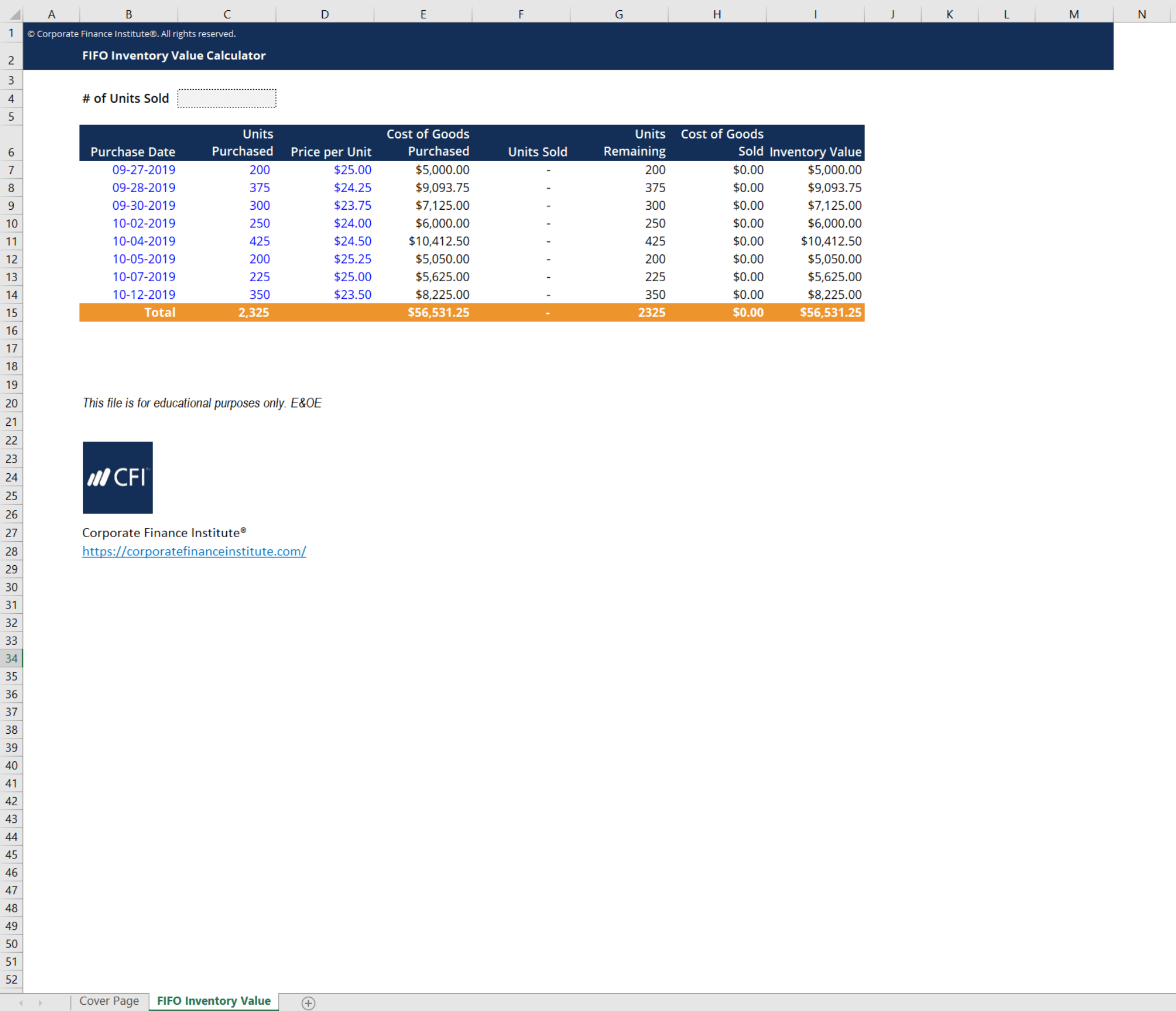# FIFO Calculator

## FIFO Calculator

This FIFO calculator will help you determine the value of your remaining inventory and cost of goods sold using the first-in-first-out method.

Here is a preview of CFI’s FIFO calculator below:### FIFO Inventory Valuation

This FIFO calculator uses the first-in-first-out method of inventory valuation to come up with an ending inventory value as well as cost of goods sold. As the name implies, this method assumes that the first inventory items that are purchased are the first ones that are pushed out for sale. A practical example of this would be a grocery store. At grocery stores, produce that comes in first is sold first, otherwise, it would perish. Thus, the most recent costs are the ones that remain on the balance sheet while older ones are expensed first.

Consider the grocery store example mentioned earlier. Here is an example of the grocery store’s schedule of apple purchases:

• June 6, 2019: 100 apples purchased at \$1.20/apple
• June 7, 2019: 100 apples purchased at \$1.45/apple
• June 9, 2019: 100 apples purchased at \$1.10/apple

If by June 10th, this grocery store sold 220 apples, what would its inventory value and COGS be?

We can calculate this by applying the FIFO method used in CFI’s FIFO calculator.

Following the schedule above, we can calculate the cost of the remaining apples and the cost of goods sold.

• June 6, 2019: 100 apples sold at \$1.20/apple = \$120 in COGS
• June 7, 2019: 100 apples sold at \$1.45/apple = \$145 in COGS
• June 9, 2019: 20 apples sold at \$1.10/apple = \$22 in COGS

Therefore, total cost of goods sold would be 120+145+22 = \$287 and the remaining inventory value would be 80*1.1 = \$88.

### More Free Templates

For more resources, check out our business templates library to download numerous free Excel modeling, PowerPoint presentation and Word document templates.

• Excel Modeling Templates
• PowerPoint Presentation Templates
• Transaction Document Templates
• CFI Template Marketplace

### Financial Analyst Certification

Become a certified Financial Modeling and Valuation Analyst (FMVA)® by completing CFI’s online financial modeling classes and training program!